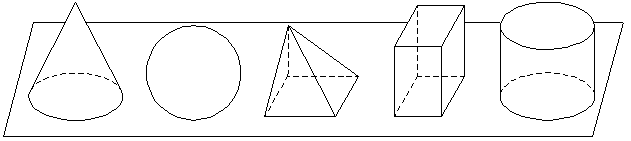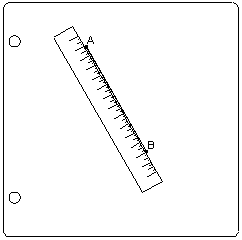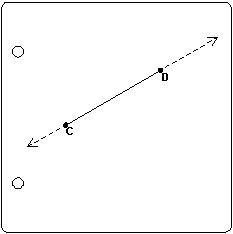CHAPTER 1
Planes, Points, and Lines

1.  You already know a great deal about geometry and geometric figures.  Since it may perhaps be difficult for you to recall these facts, we will help you by reviewing some of the material involving geometry which you have covered in your previous studies and which you have met in your everyday experiences.  First of all, we want you to test your ability to give to each of certain familiar objects the name of the geometric figure which describes the shape of that object.  To do this, we want you to use a simple matching test.

2.  Exercises:  Matching test.  In the two lists given below, match the geometric figures listed in Column II with the familiar objects listed in Column I.  For example, since a smooth-rimmed wheel is a circle, letter g is written in the parentheses next to number 1.  Also, since the flat surface of a desk is a plane, the letter i is written in the parentheses next to number 2.  Complete the test in a similar manner.

 I II 1. (g) A smooth-rimmed wheel a) Point 2. (i) The flat surface of a desk b) Line 3. (  ) The shape of the playing area of a football field c) Angle 4. (  ) The sharp end of a needle d) Right angle 5. (  ) The figure formed by the two hands of a clock at 3 o'clock e) Rectangle 6. (  ) The relation of an upright pole to the level ground f) Square 7. (  ) The four edges of the floor of a room which is just as long as it is wide g) Circle 8. (  ) A straight section of railroad track h) Perpendicular 9. (  ) The figure formed by the intersection of two spokes of a wheel i) Plane 10. (  ) The edge of a page of a book j) Parellel Lines 11. (  ) Part way around the rim of a cup k) Arc of a circle 12. (  ) A spoke of a wheel l) Radius of a circle

3. Answers to the above exercises.  To see how well you recalled the names of the geometric figures, compare your answers to the following: 1. g); 2. i); 3. e); 4. a); 5. d); 6. h); 7. f); 8. j); 9. c); 10. b); 11. k); 12. l).  Since these exercises covered only a few examples, each one of you may make up similar matching exercises using designs found on paper, on all kinds of cloth, on the walls of the room, in photographs of machinery, in advertisements, in building structures, in the designs from nature, and from other available sources.

4.  A plane.  Take a flat sheet of paper.  This flat sheet of paper represents a plane.  Of coursethe plane itself extends indefinitely beyond the four edges of the paper.  All the geometric figures with which you deal in Plane Geometry lie on a flat surface or plane.  The geometric figure which rest on a plane but which extend upward are figures used in Solid Geometry.  You are also acquainted with these figures because of your past experience.  To name only a few, you recall a cone, a sphere, a pyramid, a rectangular solid, and a cylinder.Cone, Sphere, Pyramid, Rectangular Solid, Cylinder
 5. Drawing and examining simple geometric figures:  A point and line. Again take a flat sheet of paper.  Put a dot any place on the paper and letter the dot A.  Put a second dot any other place on the paper and letter the dot B.  Let these dots represent points.  Take a ruler (or any convenient straightedge) and place it so that A and B lie along the straightedge and with a pencil draw the straight line AB.  We find that every time we draw a line from A to B along the straight edge of a ruler, the line lies exactly on AB.  So we say: Only one straight line can be drawn through two given points.6.  Postulates.  We call the above statement a postulate.  We did not prove the statement to be true, but every time we drew a straight line from A to B, we always got the same line.  So we assumed that only one straight line could be drawn from A to B.  We shall learn many postulates as the course develops, but here we will add only two more.  From the preceding figure we find:    1.  A straight line is the shortest distance between two points. And from the adjacent figure:    2.  A straight line can be extended indefinitely in either direction.7.  A line segment.  Although you know that a line can be extended, or produced, indefinitely, you deal frequently with only a portion of a line.  We call a portion of a straight line between two given points a segment or a line segment.  When the meaning in clear that we are dealing with a line segment, we often refer to it simply as a line.

8.  Size of line segments.  Often you can compare the lengths of line segments fairly accurately simply by looking at them.  Thus, in the adjacent figure, you can see that line segment a is longer than line segment b.  Sometimes this simple method will not work, for some figures are deceiving.  For example, which segment, c or d, looks the longer?  How would you find out which really is longer?  You would use your common sense and measure them.  If a ruler is handy, you measure each with a ruler.  But a more accurate method is to place one segment upon the other, a method you have used since your early childhood.  If the line segments fit exactly, we say they coincide, and are therefore equal.  Hence: Two line segments which can be made to coincide are equal to each other.

9.  How to draw a straight line segment equal in length to a given line segment.  Let us measure the given line segment AB and draw a line equal to it.   Solution:  In geometry, the compass is used to measure lines.  Open the compass so that its points rest on the ends of the given line AB.  Then place the metal point at C on line l and draw an arc with C as a center and the distance AB as a radius.  The segment CD on l equals AB.

10.  How to bisect a given line segment.  To bisect a line segment means to divide it into two equal parts.  Let us bisect the given line segment AB.  Solution:  With A as a center, and with an opening of the compass greater than one half of the length of AB, draw an arc that extends both above and below AB.  With the same radius and with B as a center, draw a similar arc.  Mark the intersections of the arcs C and D respectively.  Draw CD cutting AB at E.  Then CD bisects AB at E.  Thus we see that a line segment, such as AB, can be bisected by another line (in this case, CD), or by a point (in this case, E).  We know have the following postulates:
1.  A line segment can be bisected at only one point.
2.  Two straight lines can intersect in only one point.

11.  Notation of points and lines.  In the figures you have thus far drawn, you have labeled each point by a capital letter which is placed near the point.  Thus the notation P means point P, and Q means point Q, as in the adjacent figure.  Similarly, the notation PQ (or QP) means the line or line segment connecting points P and Q.  You have also labeled lines or line segments by placing a single small letter near the line.  Thus the notation a means the line or line segment near a, as in the adjacent figure.

12.  Exercises:  Apply Articles 4-11
1.  Point out several planes in the classroom.
2.  How is a point labeled?
3.  In what two ways may a line be labeled?
4.  What is a line segment?
5.  State two postulates concerning a straight line.
6.  By what may a line segment be bisected?
7.  In how many ways may two straight lines intersect?
8.  What is meant when we say a line segment is bisected?
9.  When are two line segments said to coincide?
10. When are two line segments said to be equal?
11. With a ruler and compass, construct a line segment equal to the line segment
12. With a ruler and compass, bisect a line segment equal to line segment